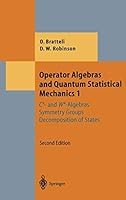#### Operator Algebras and Quantum Statistical Mechanics 1: C*- and W*-Algebras. Symmetry Groups. Decomposition of States (Theoretical and Mathematical Physics)

Operator Algebras and Quantum Statistical Mechanics 1: C*- and W*-Algebras. Symmetry Groups. Decomposition of States (Theoretical and Mathematical Physics)